library(ggplot2) # for general plotting
library(car)     # for ANOVA (Type II used, better than Type I when there is an unbalanced design)

## Information of data source

The number of eggs/mite were counted after 4 days of feeding on Moneymaker plants by 20 adult female mites of TU or TU-A on Moneymaker.

## Read in the data and view structure to identify any issues in data formatting

fecundity.data <- read.csv("~/Lab Stuff/Adapted mites/Tomato/Fecundity assay/TU-LND vs TU-Adapted LND/Tu vs Tu-A R data/Fecundity R data.csv", header = TRUE)

# Trial as a factor
fecundity.data$Trial <- factor(fecundity.data$Trial)

str(fecundity.data)
## 'data.frame':    32 obs. of  3 variables:
##  $Trial: Factor w/ 3 levels "1","2","3": 1 1 1 1 1 1 1 1 1 1 ... ##$ Mite : Factor w/ 2 levels "TU","TU-A": 1 1 1 1 1 1 2 2 2 2 ...
##  $Eggs : num 4 3.85 4.26 4.57 3.82 ... ## Formulate hypothesis H0: There will be no difference in fecundity of the mite strains. HA: TU-A will produce more eggs on Moneymaker leaflets than TU mites. ## Conduct data exploration ### Outliers in the response variable (Eggs) within explanatory variables (Trial, Mite). ggplot(fecundity.data, aes(x = Trial, y = Eggs)) + geom_boxplot() + theme_classic()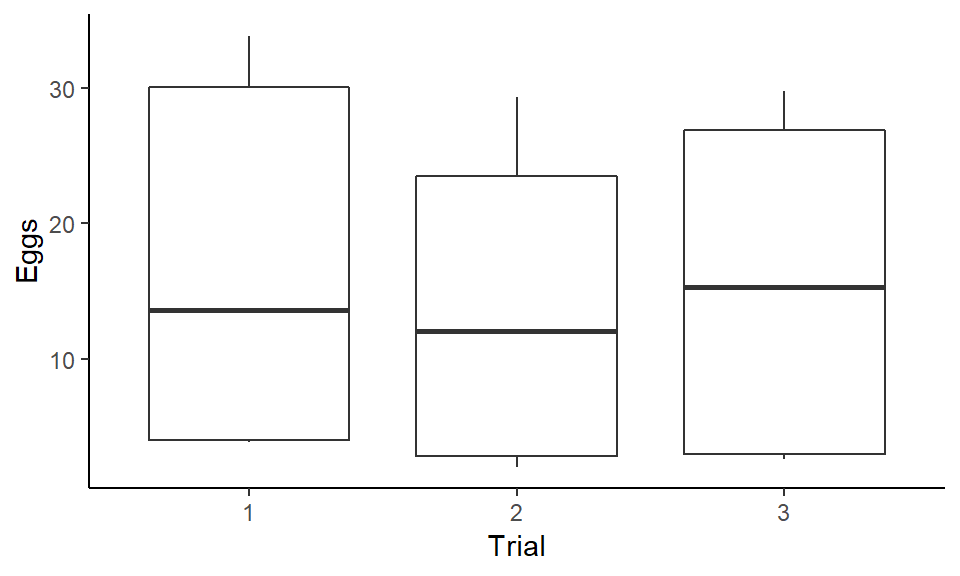ggplot(fecundity.data, aes(x = Mite, y = Eggs)) + geom_boxplot() + theme_classic()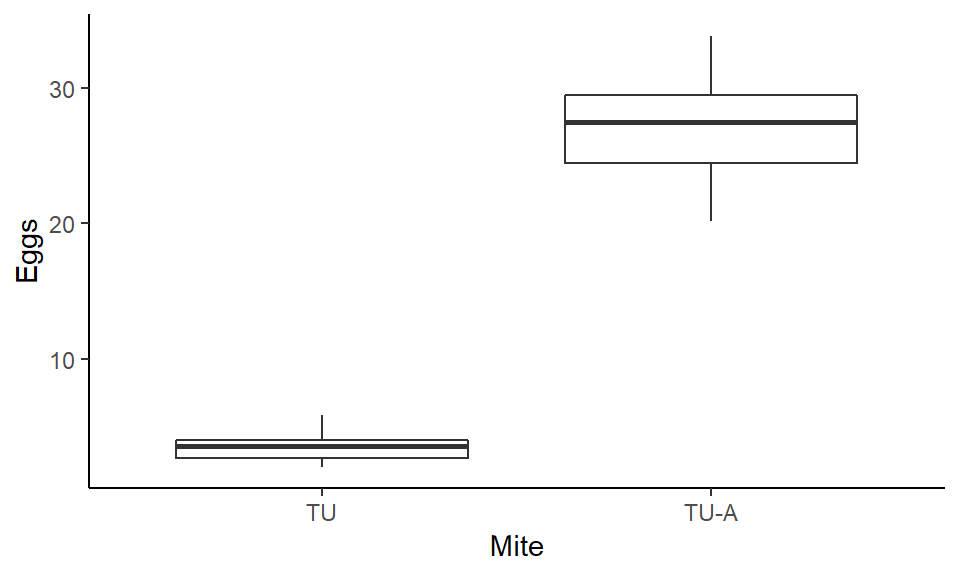No outliers. ### Collinearity of the explanatory variables Des not apply, all explanatory variables are categorical/factorial. ### Spatial/temporal or other hierarchical aspects of sampling design No, I am treating Trial as a main effect to check for reproducibility (not a random effect/blocking factor). ### Interactions (is the quality of the data good enough to include them?) Interaction betweenTrial and Mite.Strain will be performed to test for reproducibility. ### Zero inflation in Y No ### Are categorical covariates balanced? summary(fecundity.data) ## Trial Mite Eggs ## 1:12 TU :16 Min. : 2.000 ## 2:10 TU-A:16 1st Qu.: 3.697 ## 3:10 Median :12.977 ## Mean :15.329 ## 3rd Qu.:27.425 ## Max. :33.833 Not in Trial, yes in Mite. ## Apply model # fit linear model and display model fit information and ANOVA table m <- lm(Eggs ~ Mite * Trial, data = fecundity.data) summary(m) ## ## Call: ## lm(formula = Eggs ~ Mite * Trial, data = fecundity.data) ## ## Residuals: ## Min 1Q Median 3Q Max ## -6.7013 -0.8134 -0.1457 0.6802 4.5987 ## ## Coefficients: ## Estimate Std. Error t value Pr(>|t|) ## (Intercept) 4.0837 1.0967 3.724 0.000957 *** ## MiteTU-A 25.1509 1.5510 16.216 4.1e-15 *** ## Trial2 -1.2835 1.6267 -0.789 0.437219 ## Trial3 -0.6789 1.6267 -0.417 0.679858 ## MiteTU-A:Trial2 -2.9691 2.3005 -1.291 0.208185 ## MiteTU-A:Trial3 -1.6206 2.3005 -0.704 0.487401 ## --- ## Signif. codes: 0 '***' 0.001 '**' 0.01 '*' 0.05 '.' 0.1 ' ' 1 ## ## Residual standard error: 2.686 on 26 degrees of freedom ## Multiple R-squared: 0.9604, Adjusted R-squared: 0.9528 ## F-statistic: 126.2 on 5 and 26 DF, p-value: < 2.2e-16 Anova(m) ## Anova Table (Type II tests) ## ## Response: Eggs ## Sum Sq Df F value Pr(>F) ## Mite 4499.8 1 623.5359 < 2e-16 *** ## Trial 42.2 2 2.9211 0.07171 . ## Mite:Trial 12.1 2 0.8416 0.44241 ## Residuals 187.6 26 ## --- ## Signif. codes: 0 '***' 0.001 '**' 0.01 '*' 0.05 '.' 0.1 ' ' 1 # Calculate effect size and display result.anova<-Anova(m) ss<-result.anova$"Sum Sq"  ##ss = sum of squares
pes<-ss/(ss+ss[length(ss)])  ##pes = partial e squared
pes[length(pes)]<-""
result.anova$"Part E Sq"<-pes result.anova ## Anova Table (Type II tests) ## ## Response: Eggs ## Sum Sq Df F value Pr(>F) Part E Sq ## Mite 4499.8 1 623.5359 0.00000 0.95997 ## Trial 42.2 2 2.9211 0.07171 0.18347 ## Mite:Trial 12.1 2 0.8416 0.44241 0.06080 ## Residuals 187.6 26 # plot interactions interaction.plot(fecundity.data$Mite, fecundity.data$Trial, fecundity.data$Eggs, type="l", leg.bty="o", leg.bg="grey95", lwd=2, ylab="Eggs", xlab="Mite Strain", main="Mite:Trial")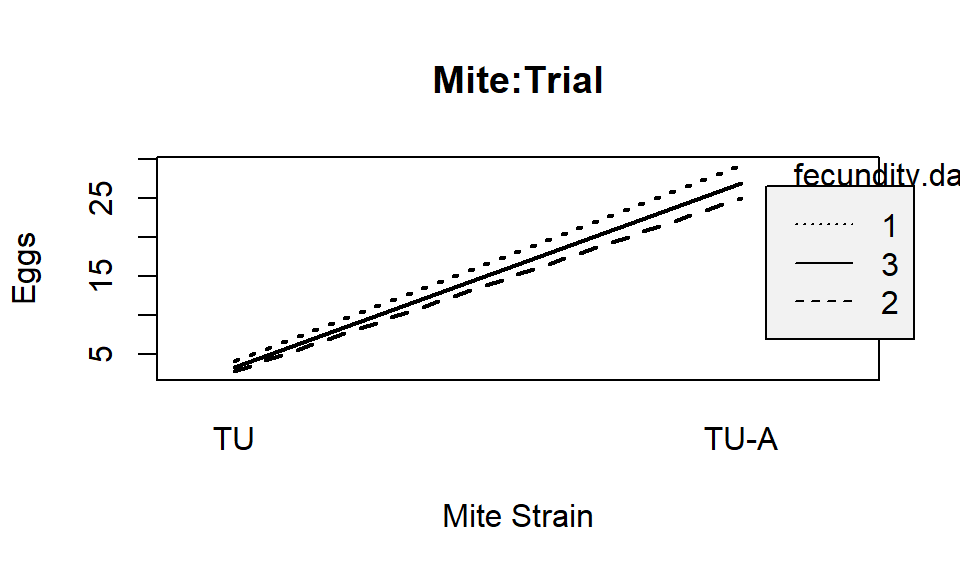## Validate model

fecundity.data$m.fit <- fitted(m) # fitted values fecundity.data$m.res <- rstandard(m) # Pearson residuals

### Residual distribution / Overdispersion

We assumed normal residuals. This is the least important regression assumption but its can be tested with a qq plot.

ggplot(fecundity.data, aes(sample = m.res)) + geom_qq() +
geom_abline(intercept = 0, slope = 1) + theme_classic()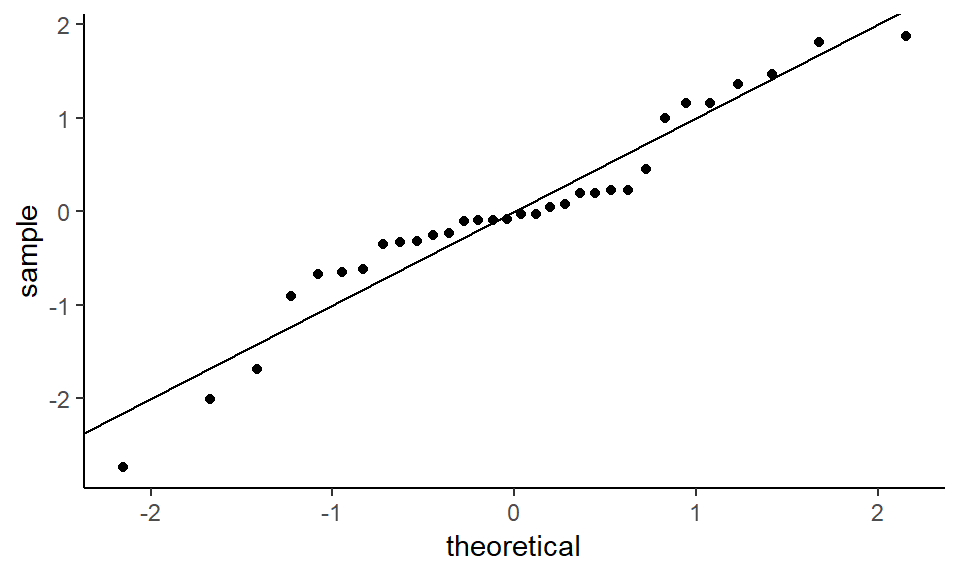Looks good.

### Residuals vs fitted values

Testing for:

Linearity - there should be no curvilinear pattern in the residuals.

Equal variance - the vertical spread of the residuals should e constant across all fitted values.

ggplot(fecundity.data, aes(x = m.fit, y = m.res)) +
geom_point() + geom_hline(yintercept = 0) + geom_smooth() + theme_classic()
## geom_smooth() using method = 'loess' and formula 'y ~ x'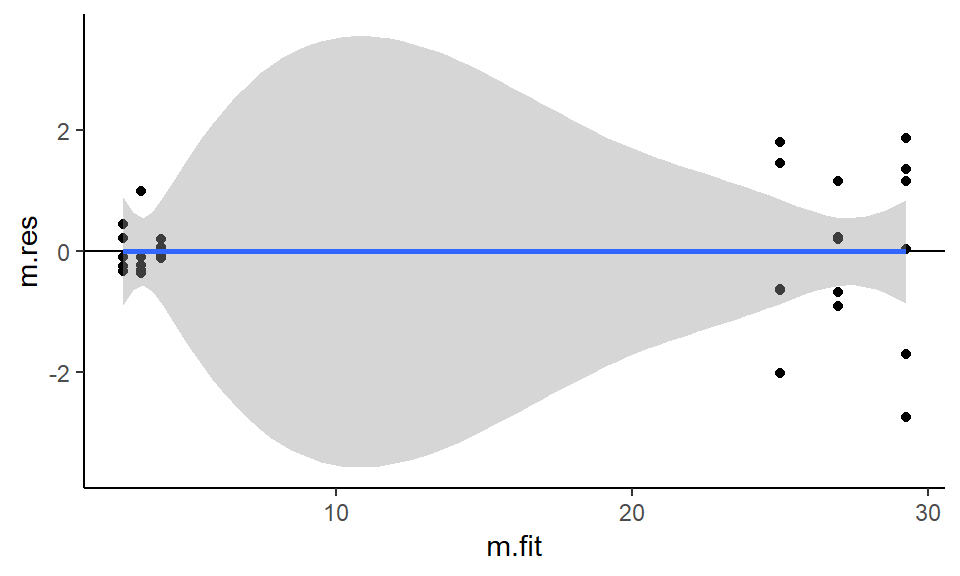Linearity - pretty good - confidence interval includes 0 for all values. Residuals farly equally dispersed.

Equal variance - no obvious problems

### Residuals vs explanatory variables

Should be centered around 0, if not then model requires another explanatory variable(s), to account for observed variation.

ggplot(fecundity.data, aes(x = Mite, y = m.res)) +
geom_boxplot() +
geom_hline(yintercept = 0) + theme_classic()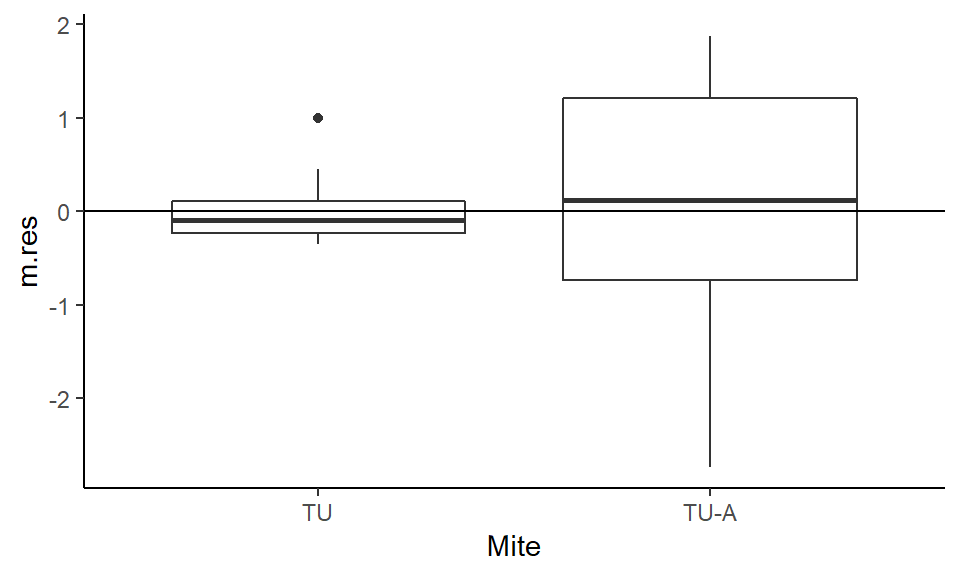ggplot(fecundity.data, aes(x = Trial, y = m.res)) +
geom_boxplot() +
geom_hline(yintercept = 0)  + theme_classic()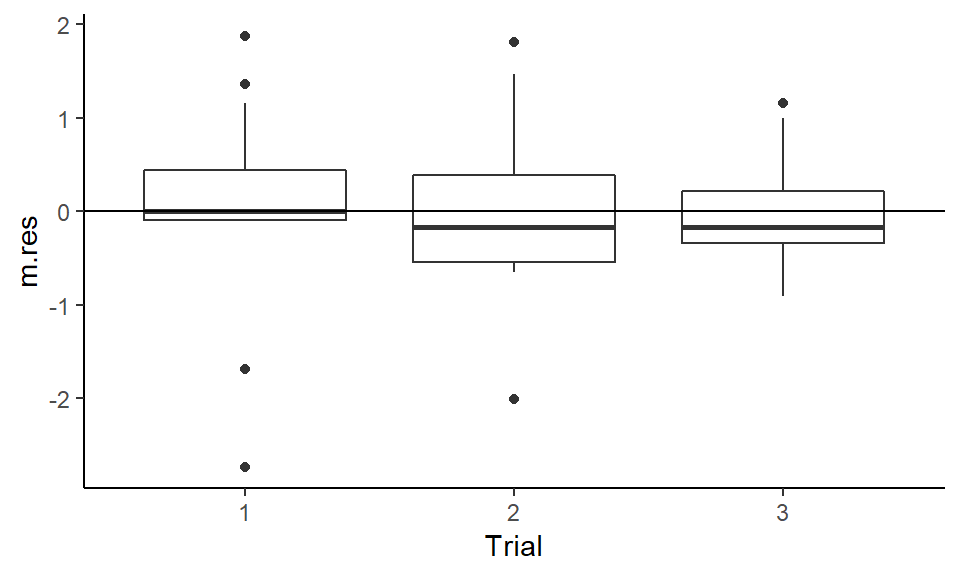ggplot(fecundity.data, aes(x = Mite:Trial, y = m.res)) +
geom_boxplot() +
geom_hline(yintercept = 0) + theme_classic()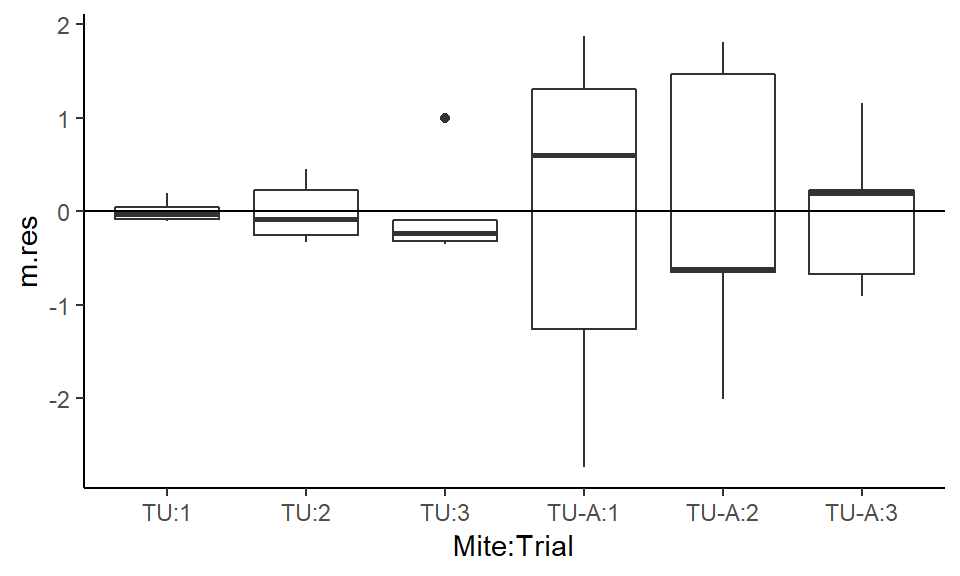Looks good.

Model is valid and interpretation of ANOVA is good.

sessionInfo()
## R version 3.6.0 (2019-04-26)
## Platform: x86_64-w64-mingw32/x64 (64-bit)
## Running under: Windows 10 x64 (build 17763)
##
## Matrix products: default
##
## locale:
##
## attached base packages:
##  stats     graphics  grDevices utils     datasets  methods   base
##
## other attached packages:
##  car_3.0-3     carData_3.0-2 ggplot2_3.1.1
##
## loaded via a namespace (and not attached):
##   zip_2.0.2         Rcpp_1.0.1        cellranger_1.1.0
##   pillar_1.4.1      compiler_3.6.0    plyr_1.8.4
##   forcats_0.4.0     tools_3.6.0       digest_0.6.19
##  evaluate_0.14     tibble_2.1.1      gtable_0.3.0
##  pkgconfig_2.0.2   rlang_0.3.4       openxlsx_4.1.0
##  curl_3.3          yaml_2.2.0        haven_2.1.0
##  xfun_0.7          rio_0.5.16        withr_2.1.2
##  stringr_1.4.0     knitr_1.23        hms_0.4.2
##  lazyeval_0.2.2    munsell_0.5.0     crayon_1.3.4